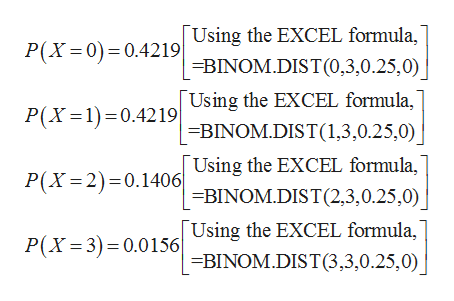# I lost my notes for solving this sample question for an upcoming exam. Some help reviewing would be awesome! Thank you! Number 7 is the one I'm looking at

Question

I lost my notes for solving this sample question for an upcoming exam. Some help reviewing would be awesome! Thank you!

Number 7 is the one I'm looking at

check_circle

star
star
star
star
star
1 Rating
Step 1

Binomial distribution:

The binomial distribution gives the probability of number of successes out of n trials in a series of Bernoulli trials.

The probability mass function (pmf) of a binomial random variable X is given as:

Step 2

Given that there are 4 possible answers for 3 multiple choice questions.

The random variable X, the numbers of questions answered correctly follows the binomial distrib...help_outlineImage TranscriptioncloseUsing the EXCEL formula, =BINOM.DIST(0,3,0.25,0) Using the EXCEL formula, P(X 0) 0.4219 P(X 1)0.4219 BINOM.DIST (1,3,0.25,0) Using the EXCEL formula, BINOM.DIST (2,3,0.25,0) Using the EXCEL formula, =BINOM.DIST(3,3,0.25,0) P(X 2) 0.1406 P(X 3) 0.0156 fullscreen

### Want to see the full answer?

See Solution

#### Want to see this answer and more?

Solutions are written by subject experts who are available 24/7. Questions are typically answered within 1 hour.*

See Solution
*Response times may vary by subject and question.
Tagged in

### Other